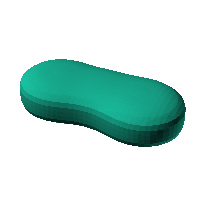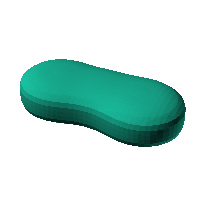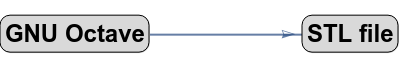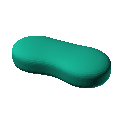Blob Farm# blob47The blob in all its glory:Think "glasses case".Click on the snapshot to download the blob's stl file.Octave Code:
```  # name of the blob
project = "blob47";

function w = f(x2,y2,z2,c,r,e)
x  = (x2-c(1))/r(1);
y  = (y2-c(2))/r(2);
z  = (z2-c(3))/r(3);
# function at origin must be <0, and >0 far enough away.  w=0 defines the surface
#    w = (x.^2+y.^2+z.^2)-1;

w = (1.0)./((x - 4).^2 + y.^2 + z.^4 + 1.) + ...
(0.3)./((x).^2 + (y - 0.5).^2 + (z*0.9).^4 + 1.) + ...
(1.0)./((x + 4).^2 + y.^2 + z.^4 + 1.) ;
w=0.11-w;
endfunction;

c_outer = [0,0,0]; # center of ellipsoid for outer surface
r_outer = [10,10,10];# x,y,z radii for ellipsoid for outer surface

step = 4;  # grid pitch in mm  start with 4mm to see the shape quickly.  Once you have it just right, change to 2mm for printing

source("../octave/func2stl_v01.m");  # do all the calculations

```
GNU Octave